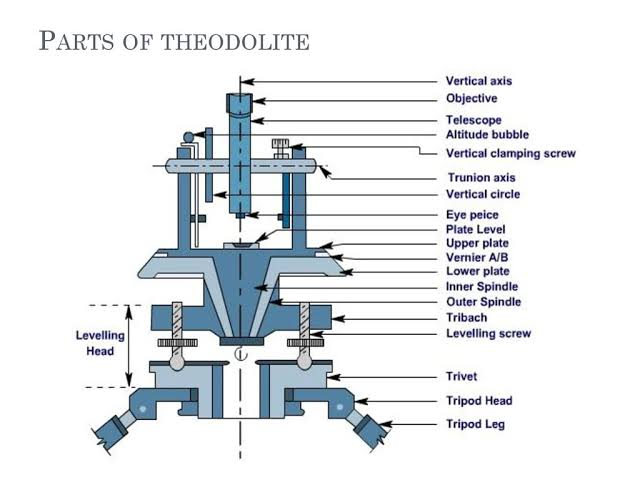HOW TO CALCULATE LOADS ON COLUMNS

The load calculation for a column depends on the type of load.

TYPES OF LOADS ON COLUMN:

Self weight of the column x Number of floors
Self weight of beams per running meter
Load of walls per running meter
The columns are also subjected to bending moments which have to be considered in the final design. The best way to design a good structure is to use advanced structural design software like ETABS or STAAD Pro. These tools are leagues ahead of manual methodology for structural design, and highly recommended.
In professional practice, there are some basic assumptions we use for structural loading calculations.

FOR COLUMNS:

Self weight of Concrete is around 2400 kg per cubic meter, which is equivalent to 240 kN. Self weight of Steel is around 8000 kg per cubic meter. Even if we assume a large column size of 230 mm x 600 mm with 1% steel and 3 meters standard height, the self weight of column is around 1000 kg per floor, which is equivalent to 10 kN. So, in my calculations, I assume self weight of column to be between 10 to 15 kN per floor.

FOR BEAMS:

Similar calculations as above. I assume each meter of beam has dimensions of 230 mm x 450 mm excluding slab thickness. So, the self weight can be around 2.5 kN per running meter.

FOR WALLS:

Density of bricks varies between 1500 to 2000 kg per cubic meter. For a 6″ thick wall of 3 meter height and a length of 1 meter, we can calculate the load per running meter to be equal to 0.150 x 1 x 3 x 2000 = 900 kg which is equivalent to 9 kN/meter. You can calculate load per running meter for any brick type using this technique.
For autoclaved, aerated concrete blocks like Aerocon or Siporex, the weight per cubic meter is between 550 to 700 kg per cubic meter. By using these blocks for construction, the wall loads per running meter can be as low as 4 kN/meter, which can result in a significant reduction in the cost of construction.

FOR SLAB:

Assume the slab has a thickness of 125 mm. Now each square meter of slab would have a self weight of 0.125 x 1 x 2400 = 300 kg which is equivalent to 3 kN. Now, assume Finishing load to be 1 kN per meter and superimposed live load to be 2 kN per meter. So, we can calculate slab load to be around 6 to 7 kN per square meter.

FACTOR OF SAFETY:

In the end, after calculating the entire load on a column, please do not forget to add in the factor of safety. For IS 456:2000, the factor of safety is 1.5.
Share:

Name

Email *

Message *

Featured Post

Theodolite

Theodolite is the most precise instrument used for measuring horizontal and vertical angles. Horizontal and vertical distances can also be...